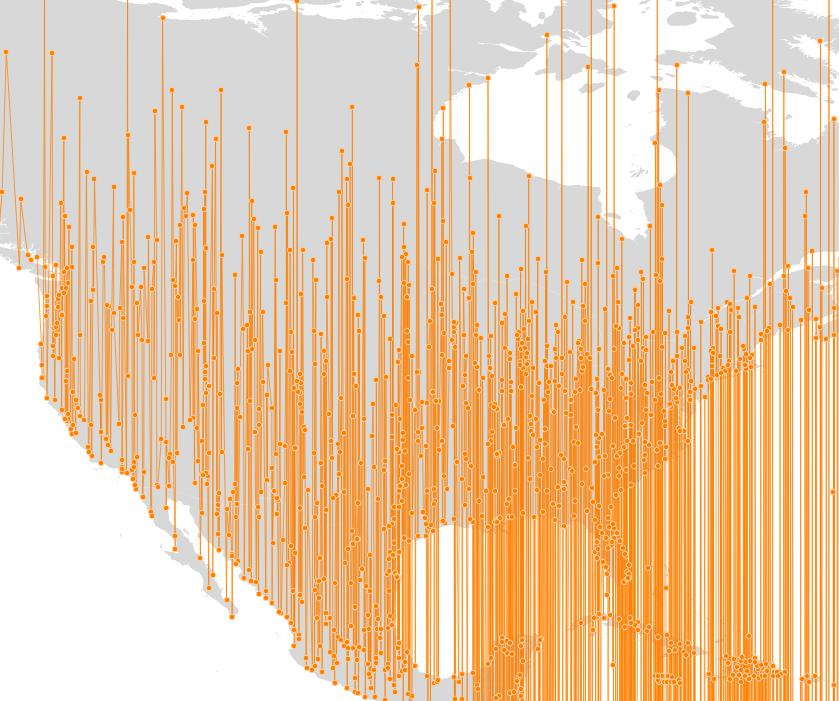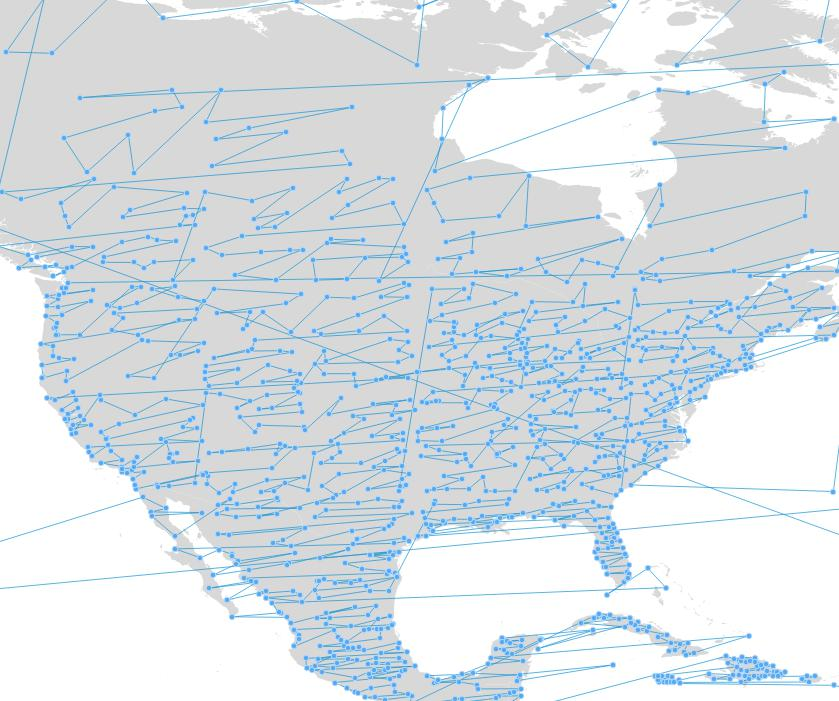# PostGIS Operators in 2.4

TL;DR: If you are using `ORDER BY` or `GROUP BY` on geometry columns in your application and you have expectations regarding the order or groups you obtain, beware that PostGIS 2.4 changes the behaviour or `ORDER BY` and `GROUP BY`. Review your applications accordingly.

The first operators we learn about in elementary school are =, > and <, but they are the operators that are the hardest to define in the spatial realm.## When is = equal?

For example, take “simple” equality. Are geometry A and B equal? Should be easy, right?1. A and B have exactly the same vertices in the same order and with the same starting points?
2. A and B have exactly the same vertices in any order? (see ST_OrderingEquals)
3. A and B have the same vertices in any order but different starting points?
4. A has some extra vertices that B does not, but they cover exactly the same area in space? (see ST_Equals)
5. A and B have the same bounds?

Confusingly, for the first 16 years of its existence, PostGIS used definition 5, “A and B have the same bounds” when evaluating the = operator for two geometries.

However, for PostGIS 2.4, PostGIS will use definition 1: “A and B have exactly the same vertices in the same order and with the same starting points”.

Why does this matter? Because the behavour of the SQL `GROUP BY` operation is bound to the “=” operator: when you group by a column, an output row is generated for all groups where every item is “=” to every other item. With the new definition in 2.4, the semantics of `GROUP BY` should be more “intuitive” when used against geometries.

## What is > and <?

Greater and less than are also tricky in the spatial domain:

• Is `POINT(0 0)` less than `POINT(1 1)`? Sort of looks like it, but…
• Is `POINT(0 0)` less than `POINT(-1 1)` or `POINT(1 -1)`? Hm, that makes the first one look less obvious…

Greater and less than are concepts that make sense for 1-dimensional values, but not for higher dimensions. The “>” and “<” operators have accordingly been an ugly hack for a long time: they compared the minima of the bounding boxes of the two geometries.

• If they were sortable using the X coordinate of the minima, that was the sorting returned.
• If they were equal in X, then the Y coordinate of the minima was used.
• Etc.

This process returned a sorted order, but not a very satisfying one: a “good” sorting would tend to place objects that are near to each other in space, near to each other in the sorted set.

Here’s what the old sorting looked like, applied to world populated places:The new sorting system for PostGIS 2.4 calculates a very simple “morton key” using the center of the bounds of a feature, keeping things simple for performance reasons. The result is a sorted order that tends to keep spatially nearby features closer together in the sorted set.Just as the “=” operator is tied to the SQL `GROUP BY` operation, the “>” and “<” operators are tied to the SQL `ORDER BY` operation. The pictures above were created by generating a line string from the populated places points as follows: